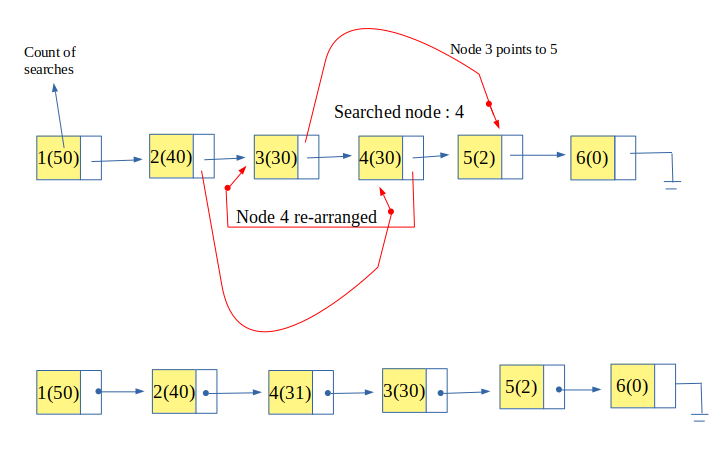Related Articles

# Self Organizing List : Count Method

• Difficulty Level : Medium
• Last Updated : 01 Jan, 2018

Self Organizing list is a list that re-organizes or re-arranges itself for better performance. In a simple list, an item to be searched is looked for in a sequential manner which gives the time complexity of O(n). But in real scenario not all the items are searched frequently and most of the time only few items are searched multiple times.

So, a self organizing list uses this property (also known as locality of reference) that brings the most frequent used items at the head of the list. This increases the probability of finding the item at the start of the list and those elements which are rarely used are pushed to the back of the list.

Attention reader! Don’t stop learning now. Get hold of all the important DSA concepts with the DSA Self Paced Course at a student-friendly price and become industry ready.  To complete your preparation from learning a language to DS Algo and many more,  please refer Complete Interview Preparation Course.

In case you wish to attend live classes with experts, please refer DSA Live Classes for Working Professionals and Competitive Programming Live for Students.

In Count Method, the number of time each node is searched for is counted (i.e. the frequency of search is maintained). So an extra storage is associated with each node that is incremented every time a node is searched. And then the nodes are arranged in non-increasing order of count or frequency of its searches. So this ensures that the most frequently accessed node is kept at the head of the list.Examples:

```Input : list : 1, 2, 3, 4, 5
searched : 4
Output : list : 4, 1, 2, 3, 5

Input : list : 4, 1, 2, 3, 5
searched : 5
searched : 5
searched : 2
Output : list : 5, 2, 4, 1, 3
Explanation : 5 is searched 2 times (i.e. the
most searched) 2 is searched 1 time and 4 is
also searched 1 time (but since 2 is searched
recently, it is kept ahead of 4) rest are not
searched, so they maintained order in which
they were inserted.
```

## Recommended: Please try your approach on {IDE} first, before moving on to the solution.

 `// CPP Program to implement self-organizing list``// using count method``#include ``using` `namespace` `std;`` ` `// structure for self organizing list``struct` `self_list {``    ``int` `value;``    ``int` `count;``    ``struct` `self_list* next;``};`` ` `// head and rear pointing to start and end of list resp.``self_list *head = NULL, *rear = NULL;`` ` `// function to insert an element``void` `insert_self_list(``int` `number)``{``    ``// creating a node``    ``self_list* temp = (self_list*)``malloc``(``sizeof``(self_list));`` ` `    ``// assigning value to the created node;``    ``temp->value = number;``    ``temp->count = 0;``    ``temp->next = NULL;`` ` `    ``// first element of list``    ``if` `(head == NULL)``        ``head = rear = temp;`` ` `    ``// rest elements of list``    ``else` `{``        ``rear->next = temp;``        ``rear = temp;``    ``}``}`` ` `// function to search the key in list``// and re-arrange self-organizing list``bool` `search_self_list(``int` `key)``{``    ``// pointer to current node``    ``self_list* current = head;`` ` `    ``// pointer to previous node``    ``self_list* prev = NULL;`` ` `    ``// searching for the key``    ``while` `(current != NULL) {`` ` `        ``// if key is found``        ``if` `(current->value == key) {`` ` `            ``// increment the count of node``            ``current->count = current->count + 1;`` ` `            ``// if it is not the first element``            ``if` `(current != head) {``                ``self_list* temp = head;``                ``self_list* temp_prev = NULL;`` ` `                ``// finding the place to arrange the searched node``                ``while` `(current->count < temp->count) {``                    ``temp_prev = temp;``                    ``temp = temp->next;``                ``}`` ` `                ``// if the place is other than its own place``                ``if` `(current != temp) {``                    ``prev->next = current->next;``                    ``current->next = temp;`` ` `                    ``// if it is to be placed at beginning``                    ``if` `(temp == head)``                        ``head = current;``                    ``else``                        ``temp_prev->next = current;``                ``}``            ``}``            ``return` `true``;``        ``}``        ``prev = current;``        ``current = current->next;``    ``}``    ``return` `false``;``}`` ` `// function to display the list``void` `display()``{``    ``if` `(head == NULL) {``        ``cout << ``"List is empty"` `<< endl;``        ``return``;``    ``}`` ` `    ``// temporary pointer pointing to head``    ``self_list* temp = head;``    ``cout << ``"List: "``;`` ` `    ``// sequentially displaying nodes``    ``while` `(temp != NULL) {``        ``cout << temp->value << ``"("` `<< temp->count << ``")"``;``        ``if` `(temp->next != NULL)``            ``cout << ``" --> "``;`` ` `        ``// incrementing node pointer.``        ``temp = temp->next;``    ``}``    ``cout << endl``         ``<< endl;``}`` ` `// Driver Code``int` `main()``{``    ``/* inserting five values */``    ``insert_self_list(1);``    ``insert_self_list(2);``    ``insert_self_list(3);``    ``insert_self_list(4);``    ``insert_self_list(5);`` ` `    ``// Display the list``    ``display();`` ` `    ``search_self_list(4);``    ``search_self_list(2);``    ``display();`` ` `    ``search_self_list(4);``    ``search_self_list(4);``    ``search_self_list(5);``    ``display();`` ` `    ``search_self_list(5);``    ``search_self_list(2);``    ``search_self_list(2);``    ``search_self_list(2);``    ``display();``    ``return` `0;``}`

Output:

```List: 1(0) --> 2(0) --> 3(0) --> 4(0) --> 5(0)
List: 2(1) --> 4(1) --> 1(0) --> 3(0) --> 5(0)
List: 4(3) --> 5(1) --> 2(1) --> 1(0) --> 3(0)
List: 2(4) --> 4(3) --> 5(2) --> 1(0) --> 3(0)
```

My Personal Notes arrow_drop_up